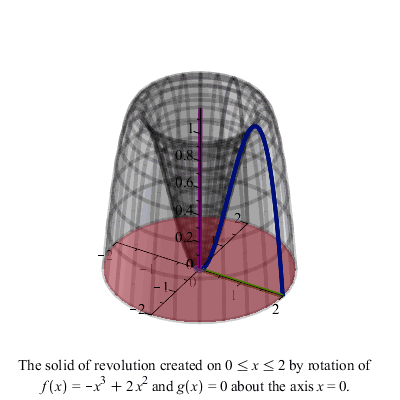# Solids of Revolution

The images on this page were all generated using a Maple worksheet.

## Disk Method

Suppose we rotate the following area around the $$x$$-axis.

We get the volume in the following animation. The volume is generated by drawing reference rectangles perpendicular to the axis of revolution and spinning them around the axis to get “disks.” The disks are shown in blue in the animation.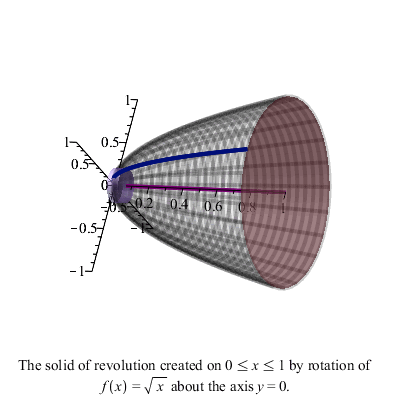## Washer Method

Suppose we rotate the following area around the $$y$$-axis.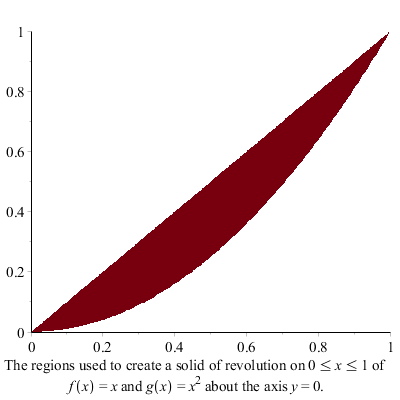We get the volume in the following animation. The volume is generated by drawing reference rectangles perpendicular to the axis of revolution and spinning them around the axis to get “washers.” The washers are shown in blue in the animation.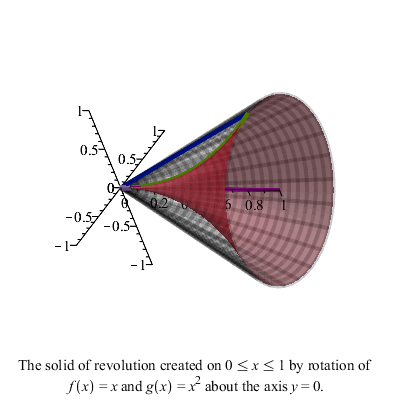## Shell Method

Suppose we rotate the following area around the $$y$$-axis.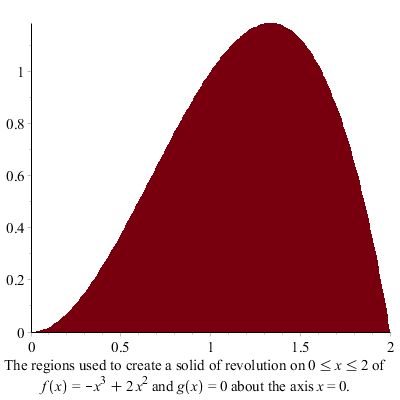We get the volume in the following animation. The volume is generated by drawing reference rectangles parallel to the axis of revolution and spinning them around the axis to get “shells.” The shells are shown in blue in the animation.